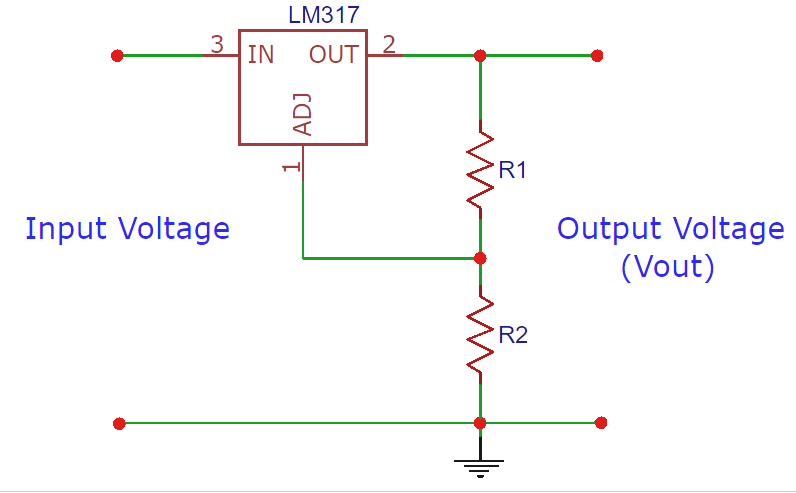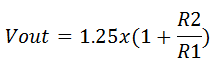# LM317 Resistor / Voltage Calculator

## The LM317 needs two external resistors — R1 and R2 — to adjust the output voltage. The output voltage depends on the values of R1 and R2.

Inputs
Resistance 1 (R1)
Resistance 2 (R2)
Output Voltage (Vout)LM317 is an adjustable voltage regulator that takes an input voltage of 3 - 40V DC and provides a fixed output voltage of 1.25V to 37V DC. It requires two external resistors to adjust the output voltage.

The output voltage Vout is dependent on external resistor values R1 and R2, according to the following equation:The recommended value for R1 is 240Ω but it can also be some other value between 100Ω to 1000Ω. So you need to enter a value of R2 in the LM317 voltage calculator to calculate the output voltage. For example, let’s take the R2 value of 1000Ω, so according to the formulae above the calculations for output voltage would be as follows:

$$Vout = 1.25x(1 + 1000/240) = 6.458V$$

Similarly, if you have a target output voltage then you can calculate the R2 value using the LM317 formulae given above. For example, if the target output voltage is 10V then the R2 value is calculated as follows:

$$10 = 1.25x(1 + R2/240)$$

$$=> R2 = 1680Ω$$

So this is how we calculate the R2 and output voltage for the LM317 voltage regulator circuit. This LM317 calculator can be also be used for some other ICs like LM338 or LM350.

### Applications

Electrical engineering calculator to find the Lm317 V and R-value. Basically, the calculation is done in this electrical engineering calculator by entering the V and R1 values to find the R2 value.